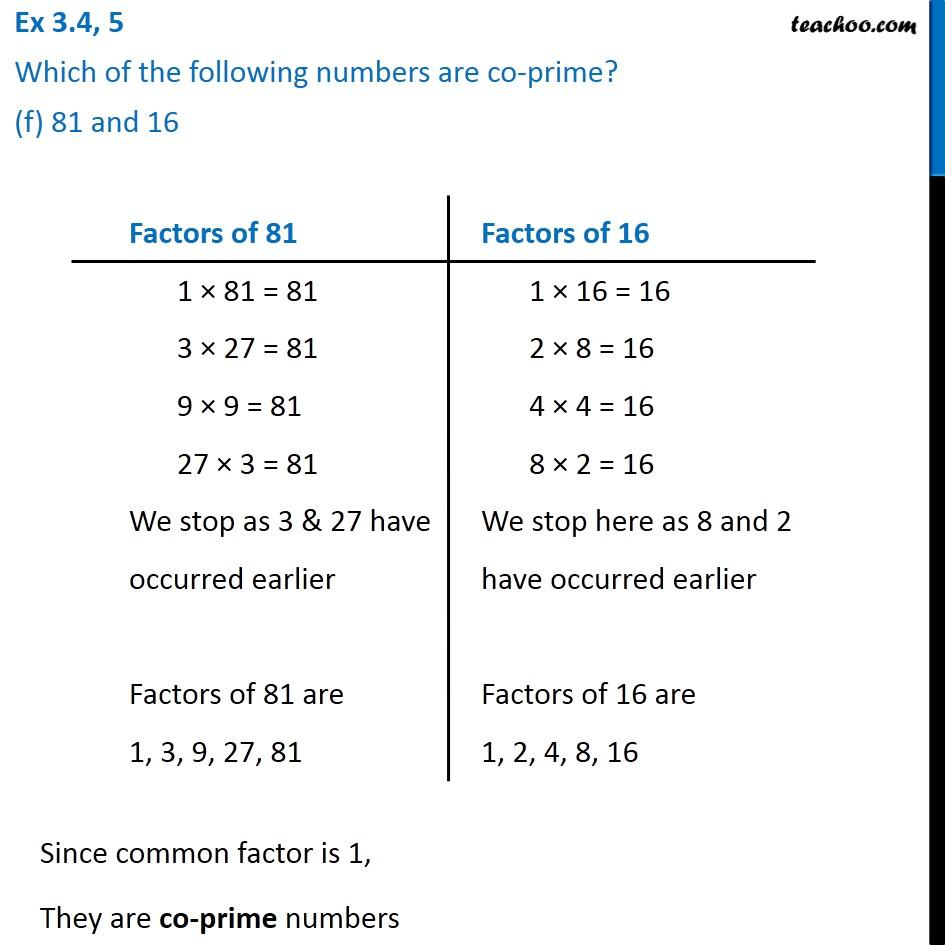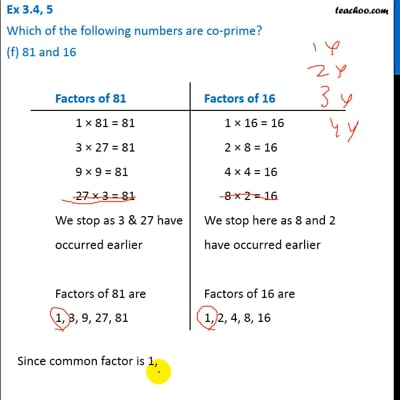Ex 3.4

Chapter 3 Class 6 Playing with Numbers
Serial order wiseThis video is only available for Teachoo black users

Introducing your new favourite teacher - Teachoo Black, at only ₹83 per month

### Transcript

Ex 3.4, 5 Which of the following numbers are co-prime? (f) 81 and 16 Factors of 81 1 × 81 = 81 3 × 27 = 17 9 × 9 = 81 27 × 3 = 81 We stop as 3 & 27 have occurred earlier Factors of 81 are 1, 3, 9, 27, 81 Factors of 16 1 × 16 = 16 2 × 8 = 16 4 × 4 = 16 8 × 2 = 16 We stop here as 8 and 2 have occurred earlier Factors of 16 are 1, 2, 4, 8, 16 Factors of 16 1 × 16 = 16 2 × 8 = 16 4 × 4 = 16 8 × 2 = 16 We stop here as 8 and 2 have occurred earlier Factors of 16 are 1, 2, 4, 8, 16 Since common factor is 1, They are co-prime numbers